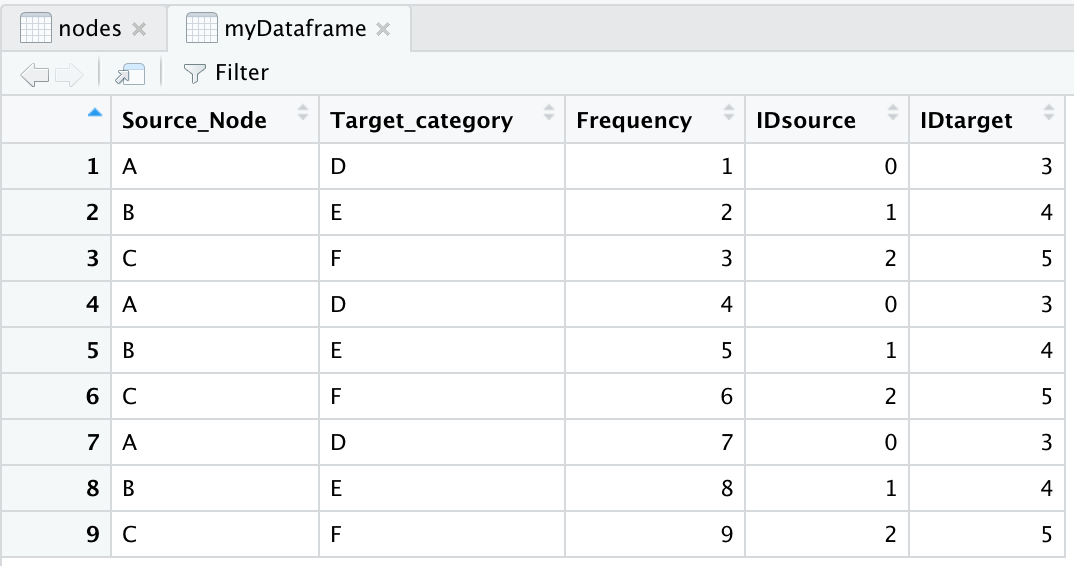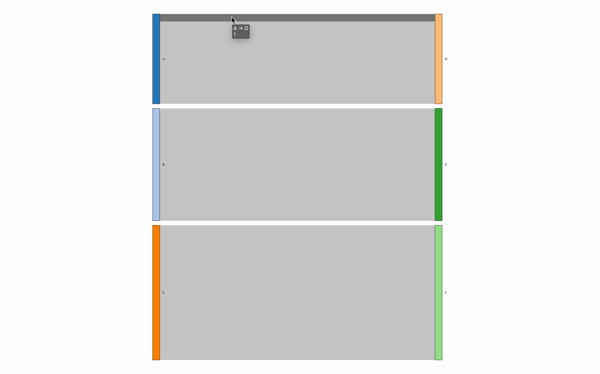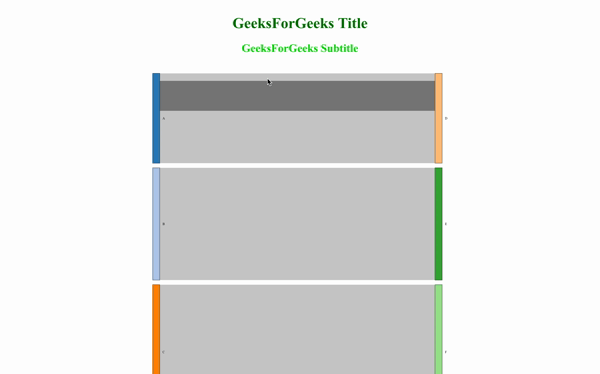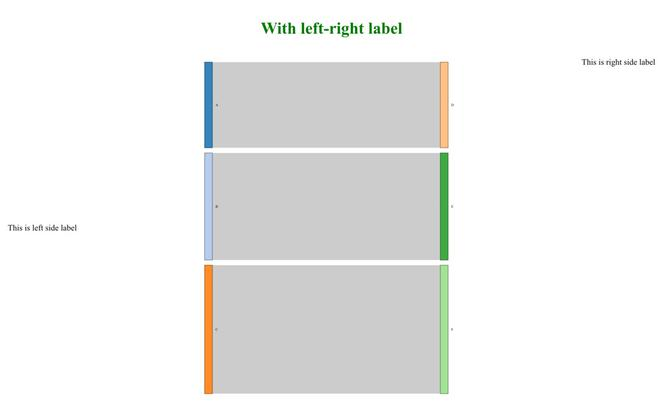Open in App
Not now

# How to add Axis labels using networkD3 in R

• Last Updated : 28 Jun, 2022

networkD3 is an R package used for creating a D3 (Data-Driven Documents) Network Graph. netwrorkD3 is constructed using the htmlwidget package. As the name said network, this graph can be constructed in the shape of a node and edge data frame. then it will perform a physics simulation to decide the most advantageous diagram in three dimensions. It is used to create a D3 Javascript network, tree, dendrogram, Sankey graph, etc.

In the R package, there are already many visualization libraries present. For example ggplot, Plotly, igraph, etc. But not all libraries are used to create network-type graphs and it is also not in 3D plots. That is the main objective behind why it is made.

Syntax: sankeyNetwork( Links, Nodes, Source, Target, Value, NodeID, NodeGroup = NodeID,                   LinkGroup = NULL, units = “”, colourScale = NULL, fontSize = 7, fontFamily = NULL, nodeWidth = 15, nodePadding = 10, margin = NULL, height = NULL, width = NULL, iterations = 32, sinksRight = TRUE )

Parameters:

• Nodes: dataframe contain node properties
• Source: character string of source node
• Target: character string of target node
• Value: character string of distance value between nodes
• NodeID: node name
• NodeGroup: character string of the nodeIDs in nodes
• Units: character string to specify physical unit(value)
• colourscale: specify color scale
• nodeWidth: width of node
• sinksRight: Boolean. If it is True, last node moves to right border of graph

## Creating a simple sankeyNetwork graph with Title, Subtitle, and Labels

Step: 1 Import the necessary library in Rstudio.

## R

 `library``(dplyr)` `library``(networkD3)` `library``(htmlwidgets)` `library``(manipulateWidget)` `library``(htmltools)`

Step: 2 Create network data in a source-target data frame format.

• Here, we are linking A->D, B->E, and C->F nodes and assigning 3 values on each link. final table will look like myDataframe table image.
• The node table is containing every node entry uniquely.
• IDsource and IDtarget is a string character form of the source and the target node.
• match function will match take two-column and create a matching table from 1. But we are subtracting by ‘-1’ so that it can start with 0th character like for A node it is 0, B node it is 1 and so on.

## R

 `# Create values` `Source_Node <- ``c``(``"A"``,``"B"``,``"C"``,``"A"``,``"B"``,` `                 ``"C"``,``"A"``,``"B"``,``"C"``)` `Target_category <- ``c``(``"D"``,``"E"``,``"F"``,``"D"``,` `                     ``"E"``,``"F"``,``"D"``,``"E"``,``"F"``)` `Frequency <- ``c``(1,2,3,4,5,6,7,8,9)` `myDataframe <- ``data.frame``(Source_Node,` `                          ``Target_category,Frequency)`   `# Node table` `nodes <- ``data.frame``(name=``c``(``as.character``(myDataframe\$Source_Node), ` `                           ``as.character``(myDataframe\$Target_category))` `                    ``%>% ``unique``())`   `# IDsource and IDtarget string character format` `myDataframe\$IDsource=``match``(myDataframe\$Source_Node, ` `                           ``nodes\$name)-1 ` `myDataframe\$IDtarget=``match``(myDataframe\$Target_category,` `                           ``nodes\$name)-1`

Output:Step: 3 Create a simple Sankey network chart.

As per the above sankeyNetwork syntax, we are assigning some parameters to generate our output.

## R

 `graph1 <- ``sankeyNetwork``(Links = myDataframe,  ` `                        ``Nodes = nodes, ` `                   ``Source = ``"IDsource"``, ` `                        ``Target = ``"IDtarget"``, ` `                   ``Value = ``"Frequency"``,` `                        ``NodeID = ``"name"``,  ` `                   ``sinksRight=``FALSE``)`   `# view graph` `graph1`

Output:SankeyNetwork Graph

Step: 4 Add Title and Subtitle in graph1.

• To assign a title, we can use the prependContent function from the htmlwidgets tool.
• Create two h1 and h2 tags with text description and style.
• prepend these tags in our above graph1 by putting all these tags together in the prependContent function.

## R

 `graph2 <- htmlwidgets::``prependContent``(graph1, ` `                                      ``htmltools::tags\$``h1``(` `                                        ``"GeeksForGeeks Title"``,` `                                      ``style="text-align:center; ` `color:``#007500; "),` `                                      ``htmltools::tags\$``h2``(` `                                        ``"GeeksForGeeks Subtitle"``,` `                                      ``style="color:``#00D100; ` `text-align:center;"))`   `# view graph` `graph2`

Output:SankeyNetwork graph with title and subtitle

Step: 5 Add left and right labels in networkGraph.

• create left-tag as leftTx and right-tag as rightTx.
• Now, to combine leftTx, rightTx and graph2, use combineWidget from manipulatewidget library.
• combineWidget take, graph, title, left-column, right-column, and row-number as parameters and combine all these instances into one.

Syntax: combineWidgets( list = NULL, nrow = NULL, ncol = NULL, title = NULL, rowsize = 1, colsize = 1, byrow = TRUE, titleCSS = “”, header = NULL, footer = NULL, leftCol = NULL, rightCol = NULL, width = NULL, height = NULL )

## R

 `# create left-right label ` `leftTx = tags\$``div``( ` `         ``style="max-width: 30vw; height: 100%; ` `                 ``display: flex; align-items: center; ` `                 ``justify-content: center;", ` `         ``tags\$``p``(``"This is left side label"``))`   `rightTx = tags\$``p``(``"This is right side label"``,` `                   ``style=``"max-width:30vw"``)`   `# final output with label. combineWidget` `# can be use by manipulateWidget.` `graph3 <- ``combineWidgets``(graph2,` `                      ``title = tags\$``h1``(``"With left-right label"``,` `                      ``style=``"text-align:center;color:#007500;"``),` `                      ``leftCol = leftTx,` `                      ``rightCol = rightTx,` `                      ``nrow = 1)`   `# view graph` `graph3`

Output:Output with left & right label

My Personal Notes arrow_drop_up
Related Articles